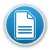I can… K 1 2 3

## Resources:

Understand that spreadsheets, are a tool used to collect, manage, analyze and visualize data. E ECreating a Spreadsheet and a Graph – A Lesson for Grades 3-7
Identify and explain terms and concepts related to spreadsheets (i.e. cell, column, row, values, labels, chart, graph) E
Enter/edit data and text into a spreadsheet and perform calculations using formulas O
Use mathematical symbol and text in a spreadsheet
Utilize spreadsheet data to create tables, charts and graphs.
Use spreadsheets to make predictions, solve problems and draw conclusions.
E = Emerging   D= Developing    P= Proficient   (O=optional) K 1 2 3 Return to Technology Skills by Grade

Click here if you have a resource that you would like to share.